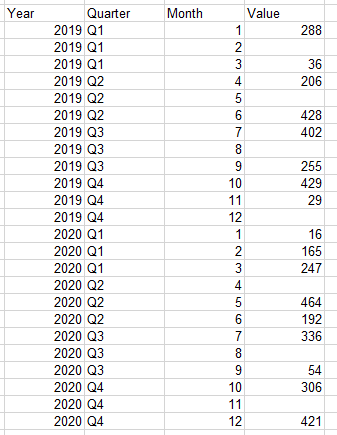# QlikView App Dev

Discussion Board for collaboration related to QlikView App Development.

Announcements
Join this live chat April 6, 10AM EST - QlikView to Qlik Sense REGISTER
cancel
Showing results for
Search instead for
Did you mean:Creator

## Max Month per Quarter in set analysis if sum(value) not null

Hello everyone,I have these datas

I would like to display the sum of value when taking into account the max month of a quarter when there is a value.

Basically for each quarter i would like the max month when value is not null

Basically it would be something like that :

if (Quarter = 'Q1' ,SUM({<CAT_CONTRAT = {'CDI'}, Annee = {'\$(=max(Year)-1)'} ,
Month = {'???????????'}>}Value),

if (Quarter = 'Q2',SUM({<CAT_CONTRAT = {'CDI'}, {'\$(=max(Year)-1)'} ,
Month = {'???????????'}>}Value),

if (Quarter = 'Q3' ,SUM({<CAT_CONTRAT = {'CDI'}, {'\$(=max(Year)-1)'} ,
Month = {'???????????'}>}Value),

if (Quarter = 'Q4' ,SUM({<CAT_CONTRAT = {'CDI'}, Annee = {'\$(=max(Year)-1)'} ,
Month = {'???????????'}>}Value),))))

Thank you

Labels (3)

• ### Set Analisys

1 Solution

Accepted SolutionsSpecialist

May be try something like

for Value

``Only(Aggr(Max({<Value={">0"},CAT_CONTRAT={'CDI'}>}Value),Quarter, Year))``

For Month Name

``````Month(Date#(Aggr(Max({<Value={">0"},CAT_CONTRAT={'CDI'}>}Month),Quarter, Year)
, 'M'))``````If this doesn't work let us know the dimensions you are using or expected output.

3 RepliesSpecialist

May be try something like

for Value

``Only(Aggr(Max({<Value={">0"},CAT_CONTRAT={'CDI'}>}Value),Quarter, Year))``

For Month Name

``````Month(Date#(Aggr(Max({<Value={">0"},CAT_CONTRAT={'CDI'}>}Month),Quarter, Year)
, 'M'))``````If this doesn't work let us know the dimensions you are using or expected output.Creator
Author

Hello,

It worked well thank you !Specialist

Glad could be of help.

---------------------------------------

``To help users find verified answers, please do not forget to use the "Accept as Solution" button on any post(s) that helped you resolve your problem or question.``